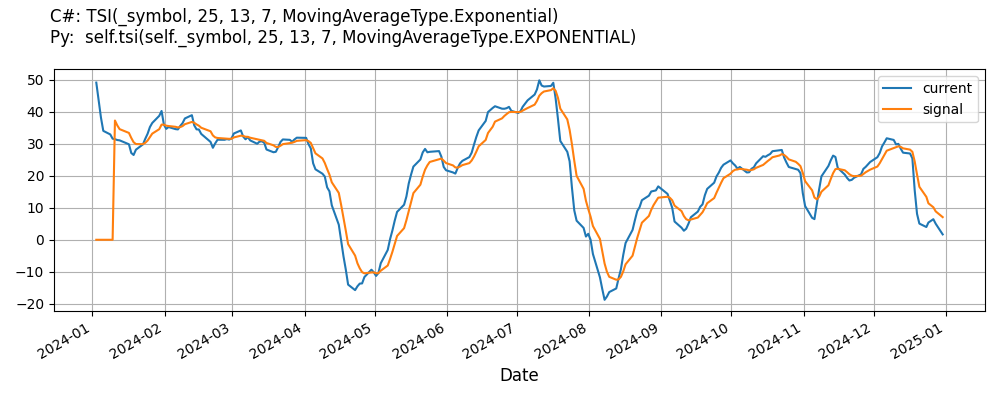# Supported Indicators

## True Strength Index

### Introduction

This indicator computes the True Strength Index (TSI). The True Strength Index is calculated as explained here: https://school.stockcharts.com/doku.php?id=technical_indicators:True_strength_index Briefly, the calculation has three steps: 1. Smooth the momentum and the absolute momentum by getting an EMA of them (typically of period 25) 2. Double smooth the momentum and the absolute momentum by getting an EMA of their EMA (typically of period 13) 3. The TSI formula itself: divide the double-smoothed momentum over the double-smoothed absolute momentum and multiply by 100 The signal is typically a 7-to-12-EMA of the TSI.

To view the implementation of this indicator, see the LEAN GitHub repository.

### Using TSI Indicator

To create an automatic indicators for TrueStrengthIndex, call the TSI helper method from the QCAlgorithm class. The TSI method creates a TrueStrengthIndex object, hooks it up for automatic updates, and returns it so you can used it in your algorithm. In most cases, you should call the helper method in the Initialize method.

public class TrueStrengthIndexAlgorithm : QCAlgorithm
{
private Symbol _symbol;
private TrueStrengthIndex _tsi;

public override void Initialize()
{
_tsi = TSI(_symbol, 25, 13, 7, MovingAverageType.Exponential);
}

public override void OnData(Slice data)
{
{
// The current value of _tsi is represented by itself (_tsi)
// or _tsi.Current.Value
Plot("TrueStrengthIndex", "tsi", _tsi);
// Plot all properties of tsi
Plot("TrueStrengthIndex", "signal", _tsi.Signal);
}
}
}
class TrueStrengthIndexAlgorithm(QCAlgorithm):
def Initialize(self) -> None:
self.tsi = self.TSI(self.symbol, 25, 13, 7, MovingAverageType.Exponential)

def OnData(self, slice: Slice) -> None:
# The current value of self.tsi is represented by self.tsi.Current.Value
self.Plot("TrueStrengthIndex", "tsi", self.tsi.Current.Value)
# Plot all attributes of self.tsi
self.Plot("TrueStrengthIndex", "signal", self.tsi.Signal.Current.Value)


The following reference table describes the TSI method:

### TSI()1/1

            TrueStrengthIndex QuantConnect.Algorithm.QCAlgorithm.TSI (
Symbol                           symbol,
*Int32                           longTermPeriod,
*Int32                           shortTermPeriod,
*Int32                           signalPeriod,
*MovingAverageType               signalType,
*Nullable<Resolution>      resolution,
*Func<IBaseData, Decimal>  selector
)


Creates a TrueStrengthIndex indicator for the symbol. The indicator will be automatically updated on the given resolution.

If you don't provide a resolution, it defaults to the security resolution. If you provide a resolution, it must be greater than or equal to the resolution of the security. For instance, if you subscribe to hourly data for a security, you should update its indicator with data that spans 1 hour or longer.

The following table describes the MovingAverageType enumeration members:

You can manually create a TrueStrengthIndex indicator, so it doesn't automatically update. Manual indicators let you update their values with any data you choose.

Updating your indicator manually enables you to control when the indicator is updated and what data you use to update it. To manually update the indicator, call the Update method with time/number pair, or an IndicatorDataPoint. The indicator will only be ready after you prime it with enough data.

public class TrueStrengthIndexAlgorithm : QCAlgorithm
{
private Symbol _symbol;
private TrueStrengthIndex _tsi;

public override void Initialize()
{
_tsi = new TrueStrengthIndex(25, 13, 7, MovingAverageType.Exponential);
}

public override void OnData(Slice data)
{
if (data.Bars.TryGeValue(_symbol, out var bar))
{
_tsi.Update(bar.EndTime, bar.Close);
}

{
// The current value of _tsi is represented by itself (_tsi)
// or _tsi.Current.Value
Plot("TrueStrengthIndex", "tsi", _tsi);
// Plot all properties of tsi
Plot("TrueStrengthIndex", "signal", _tsi.Signal);
}
}
}
class TrueStrengthIndexAlgorithm(QCAlgorithm):
def Initialize(self) -> None:
self.tsi = TrueStrengthIndex(25, 13, 7, MovingAverageType.Exponential)

def OnData(self, slice: Slice) -> None:
bar = slice.Bars.get(self.symbol)
if bar:
self.tsi.Update(bar.EndTime, bar.Close)

# The current value of self.tsi is represented by self.tsi.Current.Value
self.Plot("TrueStrengthIndex", "tsi", self.tsi.Current.Value)
# Plot all attributes of self.tsi
self.Plot("TrueStrengthIndex", "signal", self.tsi.Signal.Current.Value)


To register a manual indicator for automatic updates with the security data, call the RegisterIndicator method.

public class TrueStrengthIndexAlgorithm : QCAlgorithm
{
private Symbol _symbol;
private TrueStrengthIndex _tsi;

public override void Initialize()
{
_tsi = new TrueStrengthIndex(25, 13, 7, MovingAverageType.Exponential);
RegisterIndicator(_symbol, _tsi, Resolution.Daily);
}

public override void OnData(Slice data)
{
{
// The current value of _tsi is represented by itself (_tsi)
// or _tsi.Current.Value
Plot("TrueStrengthIndex", "tsi", _tsi);
// Plot all properties of tsi
Plot("TrueStrengthIndex", "signal", _tsi.Signal);
}
}
}
class TrueStrengthIndexAlgorithm(QCAlgorithm):
def Initialize(self) -> None:
self.tsi = TrueStrengthIndex(25, 13, 7, MovingAverageType.Exponential)
self.RegisterIndicator(self.symbol, self.tsi, Resolution.Daily)

def OnData(self, slice: Slice) -> None:
# The current value of self.tsi is represented by self.tsi.Current.Value
self.Plot("TrueStrengthIndex", "tsi", self.tsi.Current.Value)
# Plot all attributes of self.tsi
self.Plot("TrueStrengthIndex", "signal", self.tsi.Signal.Current.Value)


The following reference table describes the TrueStrengthIndex constructor:

### TrueStrengthIndex()1/2

            TrueStrengthIndex QuantConnect.Indicators.TrueStrengthIndex (
*int                shortTermPeriod,
*int                longTermPeriod,
*int                signalPeriod,
*MovingAverageType  signalType
)


Initializes a new instance of the TrueStrengthIndex class using the specified short and long term smoothing periods, and the signal period and type.

### TrueStrengthIndex()2/2

            TrueStrengthIndex QuantConnect.Indicators.TrueStrengthIndex (
string              name,
*int                shortTermPeriod,
*int                longTermPeriod,
*int                signalPeriod,
*MovingAverageType  signalType
)


Initializes a new instance of the TrueStrengthIndex class using the specified name, the short and long term smoothing periods, and the signal period and type.

### Visualization

The following image shows plot values of selected properties of TrueStrengthIndex using the plotly library.You can also see our Videos. You can also get in touch with us via Discord.